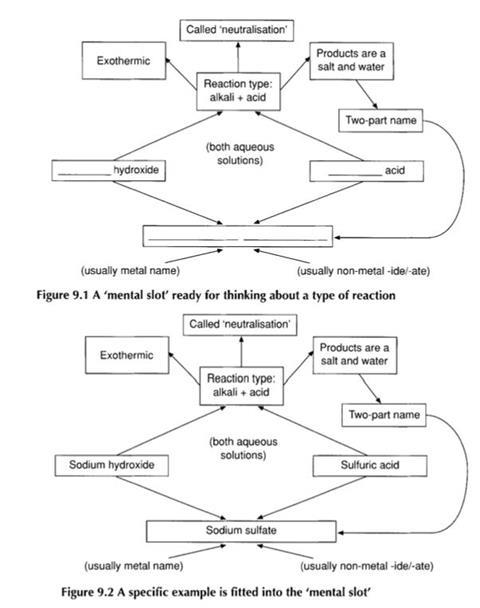The study of reactions is at the core of chemistry as a subject

The main defining characteristic of a chemical reaction is that it is a process where one or more new substances are formed.

## Describing chemical reactions

Reactants → Products

Here, the reactants and products are different chemical substances. The basic way of describing chemical reactions, then, is to write ’chemical equations’ showing the reactants and products in a particular chemical change. Chemical equations have been described as ’an essential part of the common language of scientist.

Chemical ‘equations’ are commonly written as word equations and formulae equations. There is a sense in which formulae equations are easier, as they provide a ready means of checking that no transmutation has been implied (by seeing that the same elements are represented on both sides of the equation). However, formulae equations are more abstract, and so word equations are often introduced first.

Although word equations use the (often) less abstract names of substances, rather than formulae, they can make it more difficult for students to check that the same elements are represented before and after a reaction. Students need to know, for example, that the ending ’-ate’ implies the presence of oxygen, and which elements are present in common substances such as water or ammonia.

## Student difficulties with word equations

Word equations are commonly introduced and used in lower secondary science, but national testing shows that many students find it difficult to write or complete word equations for chemical reactions.

This should not be surprising if it is remembered that although chemical names seem familiar to teachers, they may seem somewhat arbitrary to students. The more systematic names, such as tetraoxosulfate(V1) for the SO42–ion, may be especially difficult for students, if the names do not seem to fit an accessible pattern n, then students may well be concerned about the very large number of substances they could hear and read about.

For the teacher, this classification system acts as a set of familiar ’mental pigeon-holes’ into which reactions may be slotted.Students, lacking this framework for organising the information, see the equations at a much finer resolution so that a chemical equation that the teacher perceives as an integral unit seems to the students to have many components.

One of the resources included in the companion volume, Word equations, is an exercise on completing word equations. This exercise is not designed around teaching students a set of rules, but rather with giving them an opportunity to see that, although there are many possible chemical reactions, those met in school science may often be fitted into a limited number of common types.

This approach is designed to help students start constructing the reaction-type mental ’slots’ into which they can ‘drop’ reactions

## Why do reactions occur?

Explaining ‘why’ reactions occur is a much more difficult task than either completing equations or classifying reactions. Indeed, this topic is not usually dealt with in any meaningful sense until at post- 16 level. In one sense this is understandable, as valid explanations require detailed consideration of bond enthalpies, or - at least - a sophisticated application of notions of electrode potentials and Gibbs free energy. In some cases, a qualitative treatment of entropy can be used.

Yet, research has suggested that many students believe that they do know why reactions occur by the time they complete their secondary science courses. However, the reasons they commonly give are invalid, and may be in contradiction to ideas that they would be expected to learn if they continue their study of chemistry to post-1 6 level.

The most common alternative conception is to suggest that chemical reactions occur ’so that atoms can acquire a full outer electron shell’ or ’an octet of outer electrons’. Students at the end of secondary schooling, or during post-1 6 courses, will commonly give a response along these lines, even if the information given in the question clearly shows this cannot be a valid explanation.